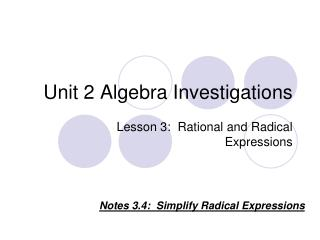DownloadDownload PresentationUnit 2 Algebra Investigations

# Unit 2 Algebra Investigations

Download Presentation## Unit 2 Algebra Investigations

- - - - - - - - - - - - - - - - - - - - - - - - - - - E N D - - - - - - - - - - - - - - - - - - - - - - - - - - -
##### Presentation Transcript

1. Unit 2 Algebra Investigations Lesson 3: Rational and Radical Expressions Notes 3.4: Simplify Radical Expressions

2. Vocabulary Radical expression: an expression containing a square root symbol. Radicand: The number or expression inside a radical symbol. Square Root: The result obtained after taking the square root of a number or expression.

3. For example: square root radical symbol radicand

4. A radical expression is in simplest form if: 1) No perfect square factors (other than 1) are in the radicand: 2) No fractions are in the radicand. 3) No radicals appear in the denominator of the fraction (the bottom part of a fraction).

5. Product Property of Radicals • The Product Property of Radicals states that the square root of a product equals the product of the square roots. • In other words, = • Examples:

6. Use the product property of radicals to rewrite the following radical expressions in their simplest form: Checkpoint: 1. 2. 3. 4. 5. 6.

7. Let’s practice multiplying radicals… We can work backwards… • = and…

8. Quotient Property The square root of a quotient (fraction) equals the quotient of the square roots of the numerator and denominator. Example:

9. Let’s practice the quotient property… Simplify the following radicals: 1) 2)

10. Checkpoint: Simplify the following radical expressions: 1. 2. 3. 4.

11. What if the denominator (the bottom part of the fraction) isn’t a perfect square???Example: In this case, we’ll multiply by the “denominator over the denominator”.

12. Checkpoint Your turn…

13. The process of eliminating a radical from an expression’s denominator is called rationalizing the denominator. In order to rationalize this type of denominator, we need to know what a conjugate is: The binomials a + and a - are called radical conjugates.

14. What is the conjugate of the following expressions: • 2) 3) 4)

15. So now we know how to find the conjugate, but how does that help us rationalize our denominator???

16. Let’s look at our previous example: First we find the conjugate, then we multiply by the “conjugate over the conjugate”… For this case, the conjugate is So we can multiply our original expression times the “conjugate over the conjugate.

17. Example:

18. Let’s look at another example:

19. Checkpoint • Simplify the following radical expressions:

20. To sum things up… How do we add or subtract radical expressions: Combine like terms. If the radicands are the same, then you can add the numbers in front of the radical sign. For example: